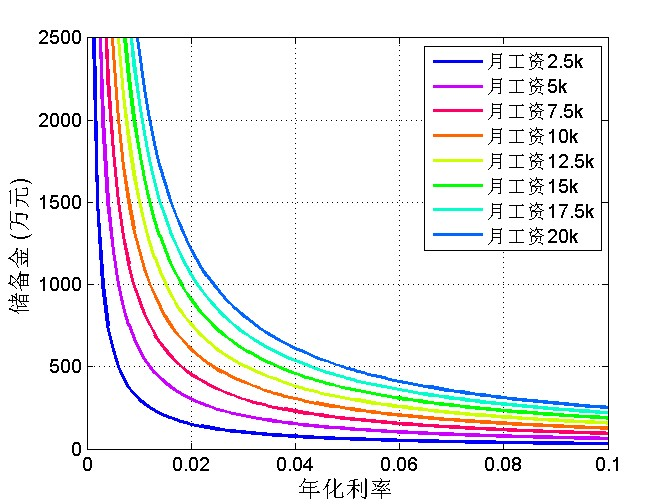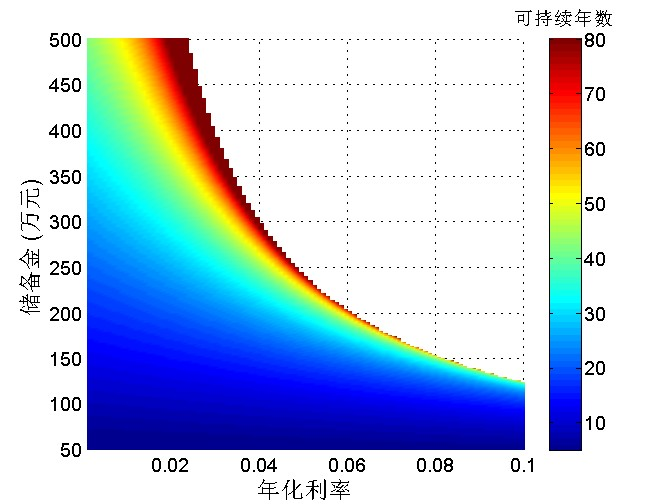# 让孩子放心地啃老的目标

4月2日的《新民晚报》“夜光杯”版面有一篇有意思的文章，讨论父母供孩子大学毕业后海外留学的支出是否必要。作者的想法大致是：本科毕业月薪5000，海归后月薪还是5000。那么干脆不要送孩子出国了，把这出国留学的100万花费剩下来给孩子发工资，让孩子堂堂正正啃老。

100万可供孩子啃老几年呢？如果不考虑利息，假设每月发给孩子5000元“工资”（啃老费），当然是200个月，也就是16.7年。那么考虑本金的利息呢？作者在文中给出的估计过于粗糙了，我们来试试严谨些的数学计算吧。

\begin{equation} P(1+j)^n=A+A(1+j)+A(1+j)^2+\cdots+A(1+j)^{n-1}=A\frac{(1+j)^n-1}{j} \end{equation}

\begin{equation} P=A\frac{(1+i)^N-1}{\left( (1+i)^{\frac{1}{12}}-1\right )(1+i)^N} \end{equation}

$N$由一个对数函数决定，如果对数的真数小于零，我们也就没法求解了。这也是最理想的情况：每个月的利息大于要发的工资，此时出不敷入，达到了诺贝尔奖金的境界。不仅孩子每个月有工资拿，父母百年之后还可以把本金原原本本留给孩子。临界公式是：

\begin{equation} (1+i)^\frac{1}{12}-1=\frac{A}{P} \end{equation}## 展开本分类索引